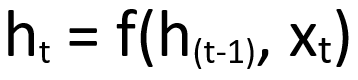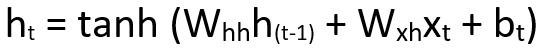Master LLMs with our FREE course in collaboration with Activeloop & Intel Disruptor Initiative. Join now!

## Publication# Traditional Recurrent Neural Networks — Reinforcement Learning Part 1/3

Last Updated on May 24, 2022 by Editorial Team

### Traditional Recurrent Neural Networks — Reinforcement Learning Part 1/3

This Blog will be a 3 part series where I will explain the different Reinforcement Learning Algorithms,

Part 1: Explanation of the Traditional Recurrent Neural Networks.
Part 2: Explanation of GRUs.
Part 3: Explanation of LSTMs.

### Introduction:

A recurrent neural network is a type of Artificial Neural Network (ANN) where the output of the previous step is fed as an input in the current step. RNN’s are primarily used in prediction problems, these can be like predicting the weather, stock market share price, or predicting the next word in a sentence given the previous words.

### How does it work ??

Before understanding how a Recurrent Neural Network works, let us understand how the weights are calculated in an RNN. The formula to calculate the weights is as below:

#### New Weights = Current Weights — (Learning rate * Gradient)

Weights: To understand we can say that these are numbers which when multiplied by inputs predict the outputs.
Learning Rate: Say we are approaching accurate predictions then learning rate tells us about how many steps are we taking towards the right solution during each iteration.
Gradient: Tells us about the direction we are moving towards the right solution.

### Intuition to understand RNN’s:

Let us see how we can relate the way we think to the way RNN works, say that you want to buy an air-cooler for your home, and you are reading the product reviews of an air-cooler. An example of the review could be something as below:

Great product, consumes less power, keeps room really cool. Would definitely suggest you buy, thumbs up.” — {1}

Thus at any point, our mind can remember words like:
Great product… less power… cool.. definitely suggest.. thumbs up..

Now if someone could ask you about the product, you would say:

It’s a great product that consumes less power, maintains the room cool, and I definitely suggest you to buy.

Now let us see how this intuition can be expressed mathematically in RNN’s.

### Mathematical working of an RNN:

In an RNN, for the sentence in {1}, the words are first converted to machine-readable vectors, then the algorithm processes these vectors one by one.

Let us have look at the representation of an RNN.

Where,
‘ht’ is the hidden state at t time step(This in our sentence example can be equivalent to the memory of the words we have, given a product review),
‘A’ is the activation (here it refers to the tanh activation),
‘xt’ is the input at ‘t’ time step,
‘t’ is the time step.

The algorithm considers the first input vector X0, processes it to produce the first hidden state h0 this becomes the input for the next layer to calculate the next hidden state h1, and so on. Thus the hidden state acts as neural network memory, it holds the data from the previous step.

Thus, the formula to calculate the hidden state given the input is as below:The equation to calculate the Hidden State (Current hidden state is a function of the previous hidden state and input).

This is further expressed with activation function as,The expanded form of calculating the hidden state with the activation function and the weights

where,
Whh is the weight at the previous hidden state,
Wxh is the weight at current hidden state,
bt is the bias.

The tanh activation function ensures that the vector of values from the hidden state remains between -1 to +1, this is because without tanh activation function certain values in the hidden state vector can become astronomically high while others remain low with information passing to the next layers.

Training Process:

1 — The input at a given timestep is provided to the network.
2 — The current state is calculated for the current input using the previous state.
3 — The current state ht becomes the hidden state h[t-1] for the next time step.
4 — One can calculate for as many time steps depending on the problem statement and join the information from previous states, however, this leads to the problem of vanishing/exploding gradient, which will be explained in my next post on LSTMs.
5 — Once all the time steps are completed the final hidden state is used to calculate the current output.
6 — The output generated is compared with the original output and the error is calculated.
7 — This error is backpropagated to improve accuracy and calculate the new weights. To know more about Backpropagation you can click here.

This is the working of an RNN, it works pretty well for short sequences and is computationally less expensive than Long Short Term Memory Networks (LSTM) or Gated Recurrent Units (GRU) networks.

### Applications of Recurrent Neural Networks:

1 — Time series anomaly detection.
2 — Music composition.
3 — Word prediction.
4 — Human Action Recognition.
5 — Predicting trends (weather prediction, share price prediction, etc)

I hope this post gives you some knowledge and motivation to further explore other topics of deep learning.

1 — If you are interested in Computer Vision, please do check the post below where I have explained how you can develop a simple code that can perform background subtraction:

OpenCV Background Subtraction and Music in Background of Video

2 — If you are interested in understanding Artificial Neural Networks and Backpropagation please do check the post below, where I have explained these concepts in simple words:

Artificial Neural Networks Simplified: From Perceptrons to BackPropagationTraditional Recurrent Neural Networks — Reinforcement Learning Part 1/3 was originally published in Towards AI on Medium, where people are continuing the conversation by highlighting and responding to this story.

Published via Towards AI